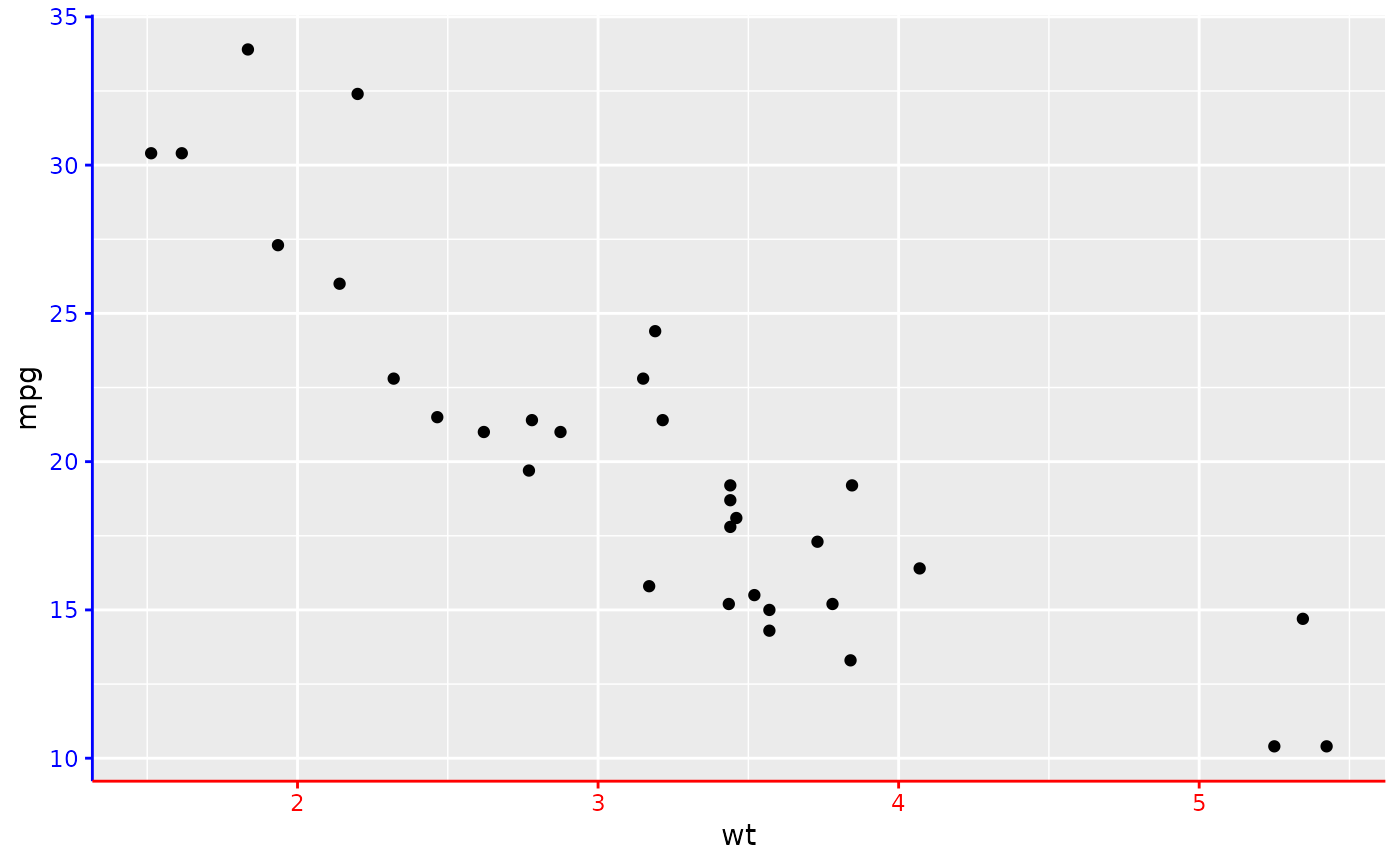This axis guide is similar to the normal axis guides for position scales, but can shorten the axis line that is being drawn. The guide_axis_colour() function is the same but with different defaults for the truncation that do not truncate the axis. Axis truncation and recolouring is supported throughout axes in ggh4x. The function is questioned due to a possible migration of guide functions after ggplot2 releases a new guide system.

## Usage

guide_axis_truncated(
title = waiver(),
check.overlap = FALSE,
angle = NULL,
n.dodge = 1,
order = 0,
colour = NULL,
color = NULL,
trunc_lower = min,
trunc_upper = max,
position = waiver()
)

guide_axis_colour(
title = waiver(),
check.overlap = FALSE,
angle = NULL,
n.dodge = 1,
order = 0,
colour = NULL,
color = NULL,
trunc_lower = NULL,
trunc_upper = NULL,
position = waiver()
)

guide_axis_color(
title = waiver(),
check.overlap = FALSE,
angle = NULL,
n.dodge = 1,
order = 0,
colour = NULL,
color = NULL,
trunc_lower = NULL,
trunc_upper = NULL,
position = waiver()
)

## Arguments

title

A character string or expression indicating a title of guide. If NULL, the title is not shown. By default (waiver()), the name of the scale object or the name specified in labs() is used for the title.

check.overlap

silently remove overlapping labels, (recursively) prioritizing the first, last, and middle labels.

angle

Compared to setting the angle in theme() / element_text(), this also uses some heuristics to automatically pick the hjust and vjust that you probably want.

n.dodge

The number of rows (for vertical axes) or columns (for horizontal axes) that should be used to render the labels. This is useful for displaying labels that would otherwise overlap.

order

Used to determine the order of the guides (left-to-right, top-to-bottom), if more than one guide must be drawn at the same location.

colour, color

A character(1) with a valid colour for colouring the axis text, axis ticks and axis line. Overrules the colour assigned by the theme.

trunc_lower, trunc_upper

The lower and upper range of the truncated axis:

• NULL to not perform any truncation.

• A function that takes the break positions as input and returns the lower or upper boundary. Note that also for discrete scales, positions are the mapped positions as numeric.

• A numeric value in data units for the lower and upper boundaries.

• A unit object.

position

Where this guide should be drawn: one of top, bottom, left, or right.

## Value

An axis_ggh4x guide class object.

Other axis-guides: guide_axis_logticks(), guide_axis_manual(), guide_axis_minor(), guide_axis_nested(), guide_axis_scalebar()

## Examples

# Make a plot
p <- ggplot(mtcars, aes(wt, mpg)) +
geom_point() +
theme(axis.line = element_line(colour = "black"))

# Setting the default truncated axis
p + guides(x = "axis_truncated")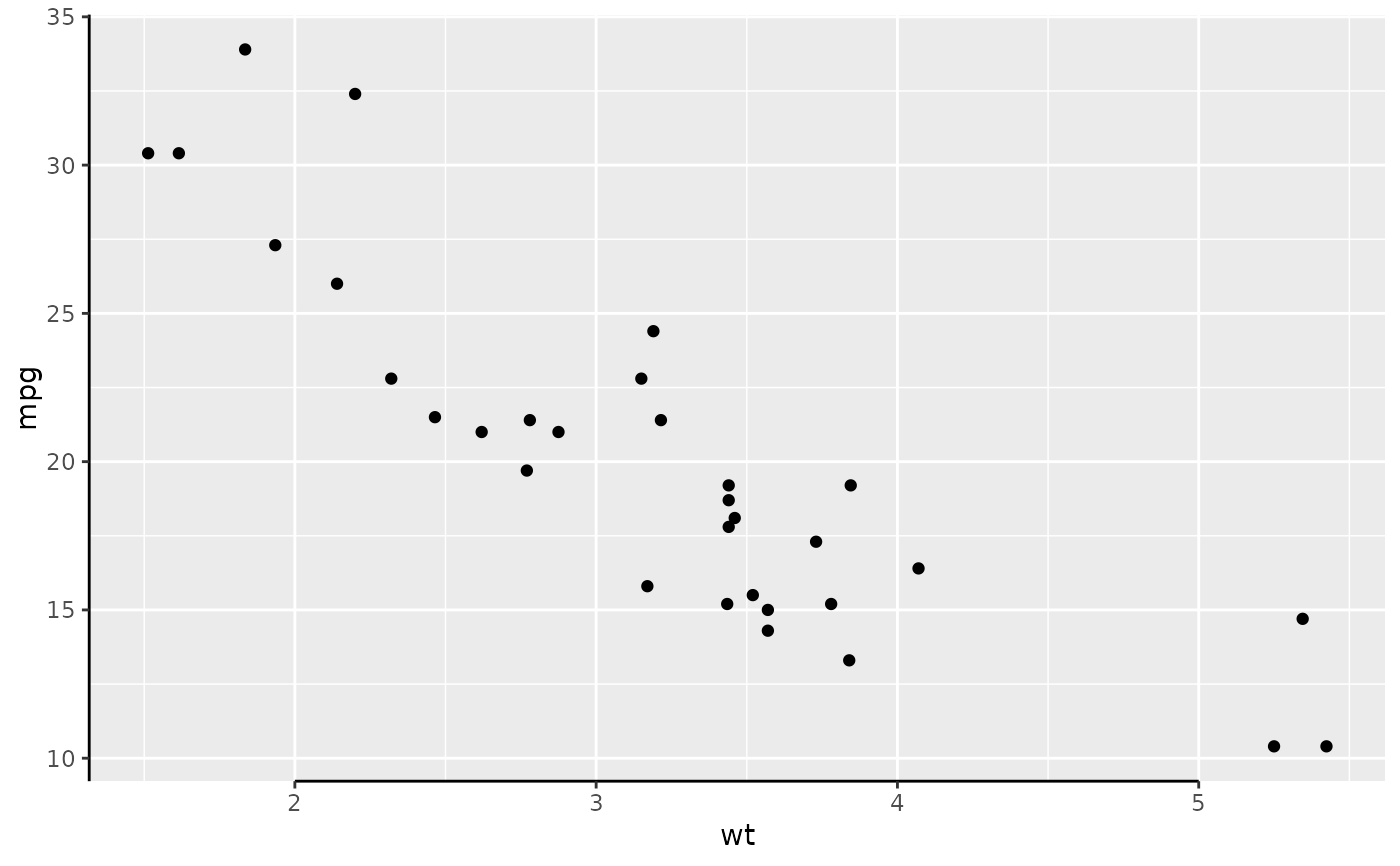# Truncating in data units
p + guides(x = guide_axis_truncated(
trunc_lower = 2.5, trunc_upper = 4.5
))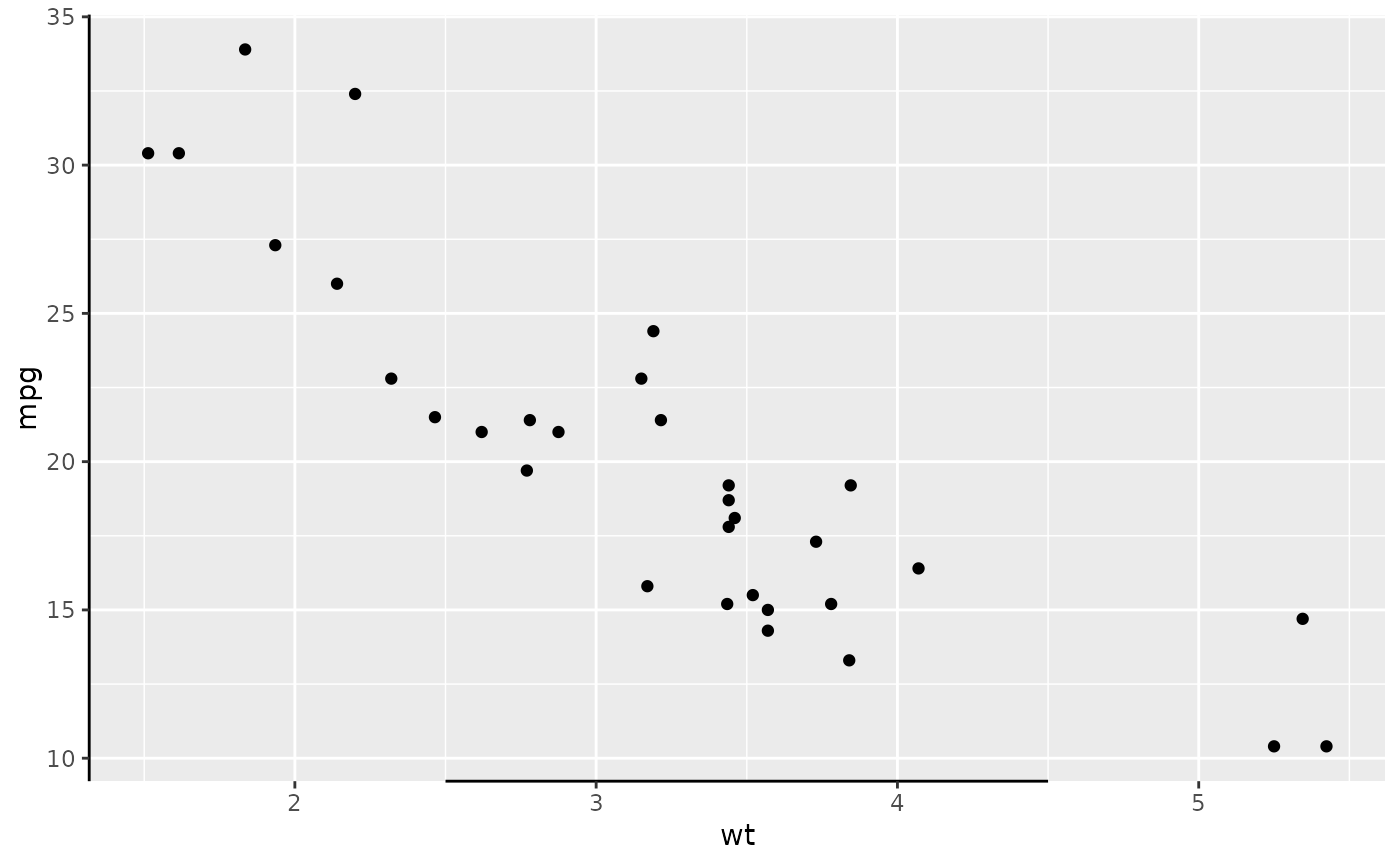# Truncate by setting units
p + guides(x = guide_axis_truncated(
trunc_lower = unit(0.1, "npc"),
trunc_upper = unit(0.9, "npc")
))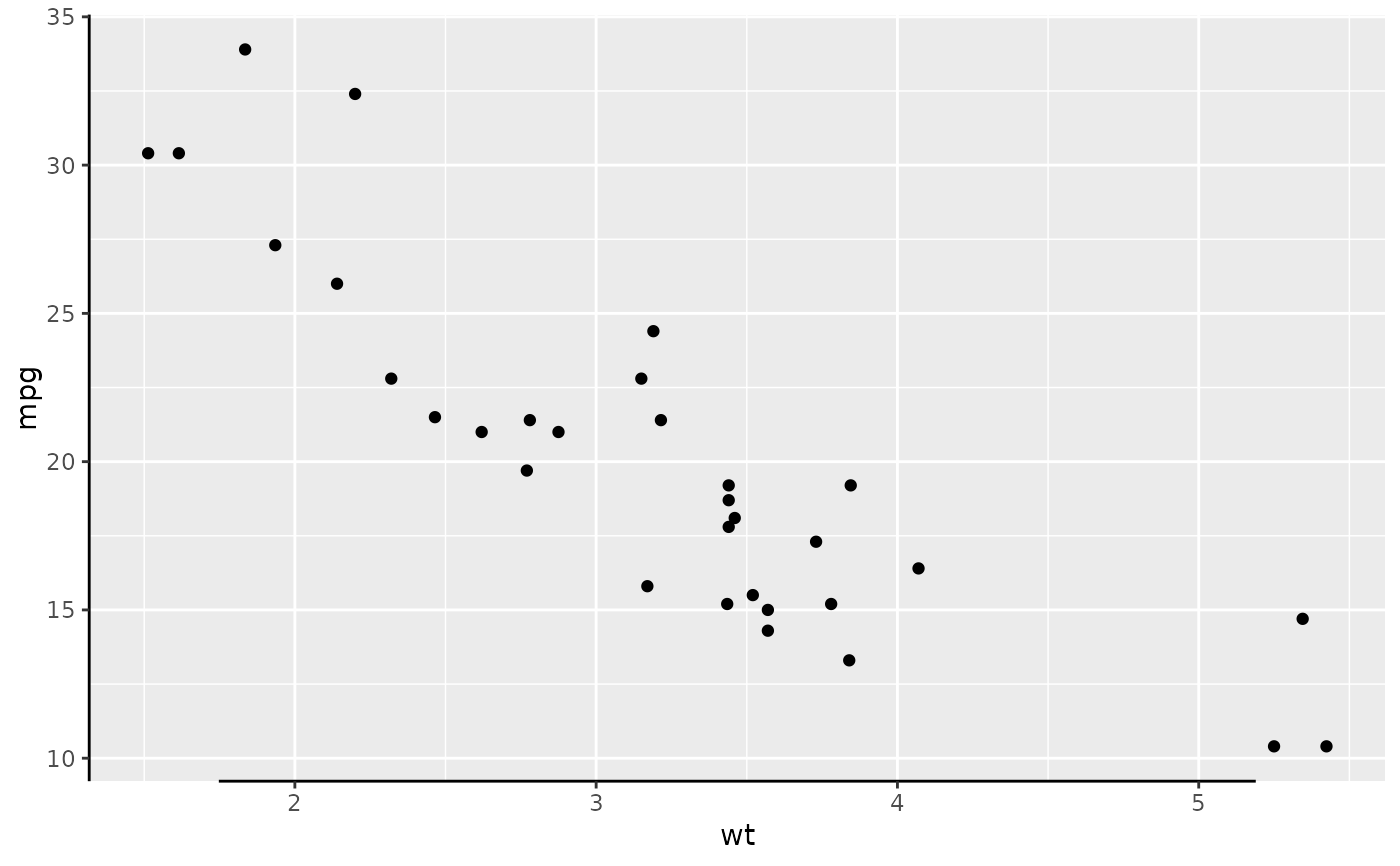# Truncating with functions
p + guides(x = guide_axis_truncated(
trunc_lower = function(x) {x - 0.2},
trunc_upper = function(x) {x + 0.2}
))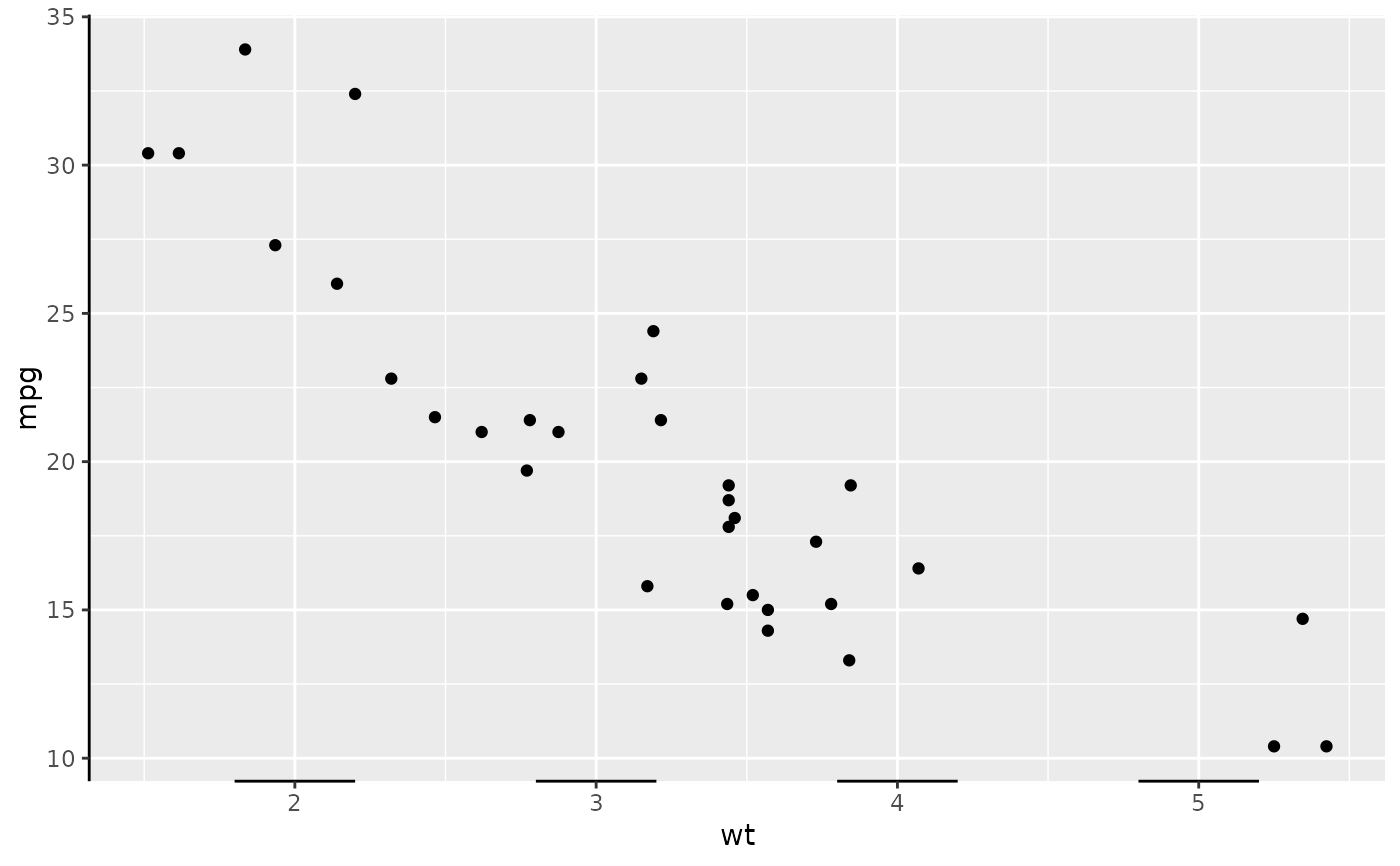# Recolouring the axes outside the theme
p + guides(x = guide_axis_colour(colour = "red"),
y = guide_axis_colour(colour = "blue"))